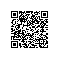# JS保留两位小数

JS保留两位小数

对于一些小数点后有多位的浮点数，我们可能只需要保留2位，但js没有提供这样直接的函数，所以我们得自己写函数实现这个功能，代码如下：

function changeTwoDecimal(x)
{
var f_x = parseFloat(x);
if (isNaN(f_x))
{
alert('function:changeTwoDecimal->parameter error');
return false;
}
var f_x = Math.round(x*100)/100;

return f_x;
}

功能：将浮点数四舍五入，取小数点后2位

用法：changeTwoDecimal(3.1415926) 返回 3.14

changeTwoDecimal(3.1475926) 返回 3.15

js保留2位小数（强制）

对于小数点位数大于2位的，用上面的函数没问题，但是如果小于2位的，比如：

changeTwoDecimal(3.1)，将返回 3.1，如果你一定需要3.10这样的格式，那么需要下面的这个函数：

function changeTwoDecimal_f(x)
{
var f_x = parseFloat(x);
if (isNaN(f_x))
{
alert('function:changeTwoDecimal->parameter error');
return false;
}
var f_x = Math.round(x*100)/100;
var s_x = f_x.toString();
var pos_decimal = s_x.indexOf('.');
if (pos_decimal < 0)
{
pos_decimal = s_x.length;
s_x += '.';
}
while (s_x.length <= pos_decimal + 2)
{
s_x += '0';
}
return s_x;
}

功能：将浮点数四舍五入，取小数点后2位，如果不足2位则补0,这个函数返回的是字符串的格式

用法：changeTwoDecimal(3.1415926) 返回 3.14

changeTwoDecimal(3.1) 返回 3.10使用钉钉扫一扫加入圈子
+ 订阅